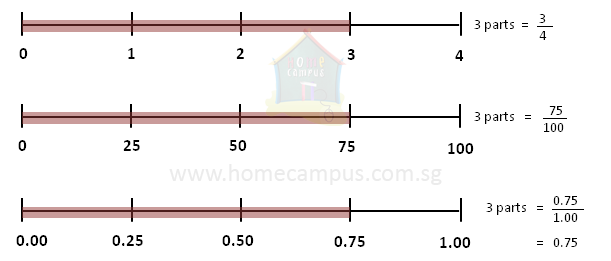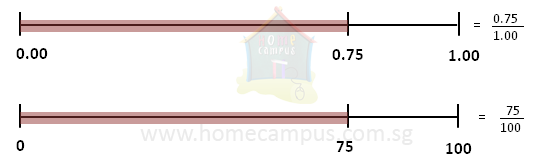## Fractions and Decimals

Practice Unlimited Questions

#### 2. Convert 3 4 to a decimal number.3 4

 3 x 25 4 x 25

 75 100

=  75  ÷  100

0.75
Change the fraction to a decimal fraction.

A decimal fraction is a fraction in which the denominator is a number related to 10.

Example: 10, 100, 1000, 10000; it's basically 1 followed by any number of zeroes.
The fraction
 3 4
is equal to the decimal number 0.75.

#### 3. Convert 0.75 to a fraction.0.75

 0.75 1

 0.75 x 100 1.00 x 100

 75 15 3 100 20 4

 3 4

The decimal number 0.75 is equal to the fraction
 3 4
.

#### 4. Change 2.625 to a fraction.

2.625

=  2  +  0.625

=  2  +
 0.625 1

=  2  +
 625 125 5 1000 200 8

=  2  +
 5 8

 2 5 8

The decimal number 2.625 is equal to the fraction
 2 5 8
.